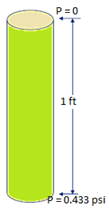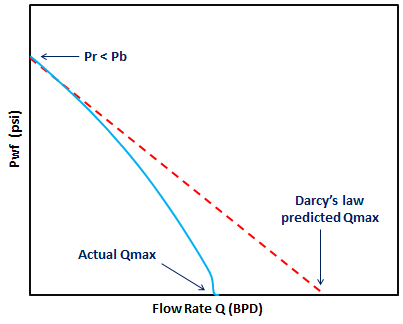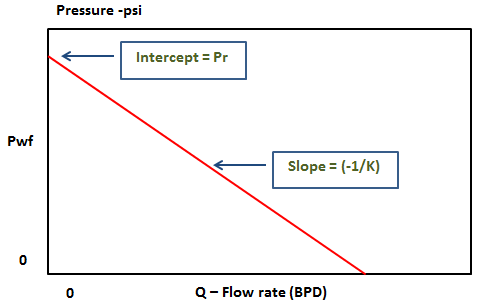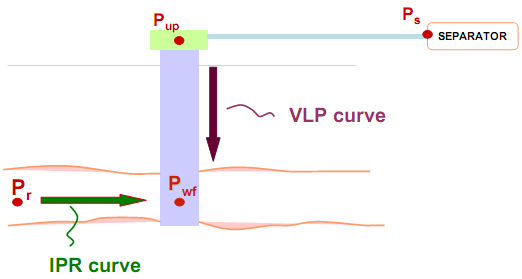### Pressure and depth:

“Pressure and Depth” is the FUNDAMENTAL relationship in the oil industry. Your understanding of the concept is crucial. The easiest way to calculate pressure from depth is to use the pressure gradient of the given fluid.

Pressure gradients for incompressible fluids have units of pressure/depth. For example, psi/ft, bar/m.

Pressure gradient seems difficult, but it is simply using the density of the fluid and converting units:

The density of pure water is 1000 kg/m3. To convert to gradient:• 1 kg = 2.2 pounds
• 1 m = 39.37 inches
• 1 m = 3.28 feet0.433 is the gradient for pure water (SG = 1) in Imperial units, remember it.

NB: Specific Gravity is always relative to pure water.

## Vogel’s inflow performance relationship

In 1968, Vogel established an empirical relationship ( Vogel’s inflow performance relationship )for flowrate prediction of a solution gas-drive reservoir in terms of the wellbore pressure based on reservoir simulation results.

### Vogel’s Model compared to PI Model…

The PI model works very well for single phase fluid (water, oil, or water/oil) flowing into a wellbore, even though water and oil are two separate phases, they are considered as a single phase since they are both liquid.

But what happens when gas comes out of solution?

” The flow velocity of a fluid in a porous medium is inversely proportional to the fluid viscosity. Typically, gas viscosity in the reservoir is about fifty times smaller than for liquid oil and consequently, the gas flow velocity is much greater. As a result, it is normal, when producing from a reservoir in which there is a free gas saturation, that gas will be produced in disproportionate amounts in comparison to the oil “. (Dake, fundamentals of reservoir engineering).

Compared to liquid, gas has much higher permeability and much lower viscosity. These two factors will give the gas a much higher flow rate than liquid inside the reservoir, so that:

QG >> QL

Below the bubble point pressure, the solution gas escapes from the oil and become free gas. The free gas occupies some portion of pore space and reduces flow of oil. This effect is quantified by:

• A decrease of oil Relative permeability
• An increase of oil viscosity (as its solution gas content drops)

Therefore, the combination of the relative permeability effect and the viscosity effect results in lower oil production rate at a given bottom-hole pressure.

Graphically, it would looks like this:## Introduction to IPR and VLP

The productivity of the well depends on an efficient use of the compressional energy available in the reservoir allowing the reservoir fluids to flow toward the production separator. As an introduction to IPR and VLP, this article will introduce two key relationships (IPR and VLP) used in the design of the production system.

### Introduction to IPR and VLP:

• Inflow Performance Relationship (IPR) is defined as the well flowing bottom-hole pressure (Pwf) as a function of production rate. It describes the flow in the reservoir. The Pwf is defined in the pressure range between the average reservoir pressure and atmospheric pressure. A typical inflow performance relationship is presented in the following graph:The intersection of the PI plot with the x-axis is the flow rate corresponding to a Pwf equal to zero. This point in the IPR plot is known as the Absolute Open Flow (AOF) potential of the well.

The following video shows more details about reservoir inflow performance:

• Vertical Lift Performance Relationship (VLP), named also Outflow, describes the bottom-hole pressure as a function of flow rate. The VLP depends on many factors including fluid PVT properties, well depth, tubing size, surface pressure, water cut and GOR. It describes the flow from the bottom-hole of the well to the wellhead.